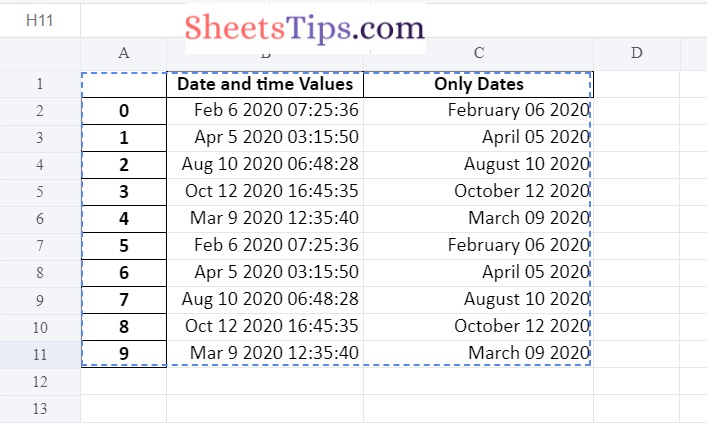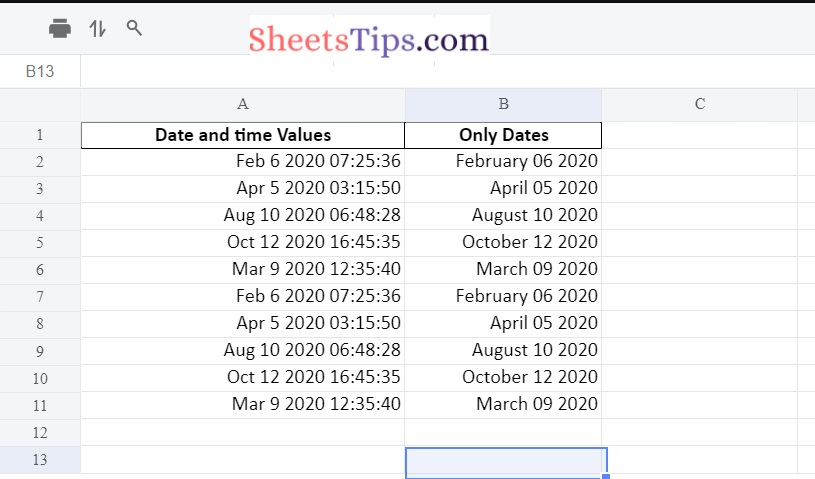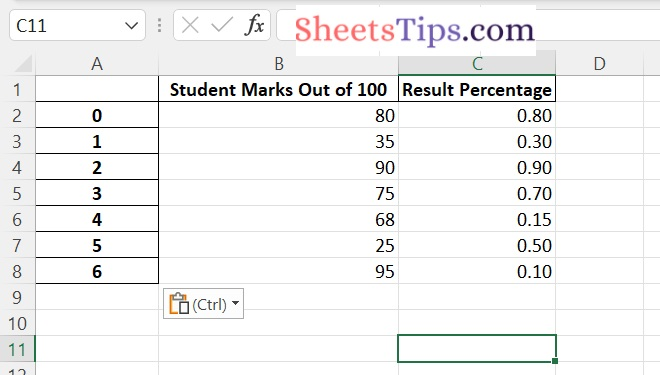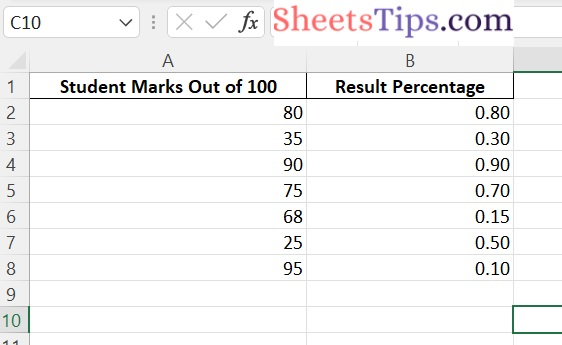# Working with Pandas and XlsxWriter in Python | Set – 2

Pandas is a data analysis library written in Python. It can read, filter, and re-arrange small and large datasets in a variety of formats, including Excel.

Pandas use the XlsxWriter modules to write Excel files.

XlsxWriter Module in Python

XlsxWriter is a Python module that allows you to write files in the XLSX file format. It can be used to fill several spreadsheets with text, numbers, and formulas. It also allows formatting, images, charts, page setup, auto filters, conditional formatting, and many more features.

Installation:

Use the following command to install the XlsxWriter module.

`pip install xlsxwriter`
Output:
```Looking in indexes: https://pypi.org/simple, https://us-python.pkg.dev/colab-wheels
/public/simple/
Collecting xlsxwriter
|████████████████████████████████| 149 kB 5.3 MB/s
Installing collected packages: xlsxwriter
Successfully installed xlsxwriter-3.0.3```

#### DataFrame.to_excel() in Pandas:

Using the to_excel() function, we can export the DataFrame to an Excel file.

The target file name must be specified when writing a single object to an excel file. If we wish to write to many/multiple sheets, we must first create an ExcelWriter object with the target filename and then indicate the sheet in the file we want to write to.

The unique sheet_name can also be used to write multiple sheets. All modifications made to the data written to the file must be saved.

NOTE:

```If we create an ExcelWriter object with a file name that already exists,
it will erase and overwrite the existing file's content.
```

Syntax:

```DataFrame.to_excel(excel_writer, sheet_name='Sheet1', na_rep='', float_format=None,
columns=None, header=True, index=True, index_label=None, startrow=0, startcol=0,
engine=None, merge_cells=True, encoding=None, inf_rep='inf', verbose=True,
freeze_panes=None)```

Parameters

• excel_writer: It is a path of an Excel file or an existing ExcelWriter.
• sheet_name: It is the name of the sheet containing the DataFrame.
• na_rep: It is the Missing Data representation.
• float_format: This is optional. It formats the string for floating-point numbers. (ex: float_format= “%.2f”, formats 0.1316 to 0.13)
• columns: These are the columns to write.
• header: The header argument writes out the column names. Suppose, If a list of the string is given, it is assumed to be the aliases for the column names.
• index: This is Boolean. If it is set to True, it writes the index else NOT.
• index_label: It indicates the column label for the index column. If it is not given and both the header and the index are True, then the index names are used. If DataFrame uses MultiIndex, a sequence must be specified.
• startrow: The default value is 0. The upper left cell row to dump the DataFrame.
• startcol: The default value is 0.  The upper left cell column to dump the DataFrame.
• engine: This is optional. It Writes the engine to use, ‘openpyxl’ or ‘xlsxwriter’. This can also be set using the options io.excel.xlsx.writer, io.excel.xls.writer, and io.excel.xlsm.writer.
• merge_cells: This is a Boolean value. The default value is True. It is used to write MultiIndex and Hierarchical rows as the merged cells.
• encoding: This is optional. It encodes the output excel file. It is just required for the xlwt.
• inf_rep: This is optional. The default value is `inf`. In most cases, it represents infinity.
• verbose: This is a Boolean value. The default value is True. It Displays more information in the error logs.
• freeze_panes: This is optional. It represents the one-based bottommost row and rightmost column that is to be frozen.

## Working with Pandas and XlsxWriter in Python

### Method #1: Using Pandas and XlsxWriter to Convert a Pandas dataframe with datetimes to an Excel file with a default datetime and date format

Approach:

• Import pandas module using the import keyword.
• Import datetime and date functions from datetime module using the import keyword.
• Create a Pandas dataframe by passing some random datetime data to the DataFrame() function of the pandas module and store it in a variable.
• datetime() function accepts five arguments (year,month,date,hour,minute,second).
• date() function accepts three arguments (year,month,date).
• Create a Pandas Excel writer object with the engine XlsxWriter.
• Set the default datetime and date format as well.
• format mmmm dd yyyy => month date year
• month – full name, date – 2 digit, year – 4 digit
• mmm d yyyy hh:mm:ss => month date year hour: minute: second
• month – first 3 letters , date – 1 or 2 digit , year – 4 digit.
• Pass the excel file path, XlsxWriter as the engine, datetime and date formats as arguments to the ExcelWriter() function of the pandas module to create a Pandas Excel writer object and store it in a variable.
• Write the above dataframe to the worksheet Object using the to_excel() function by passing the writer object and sheet Name to it.
• Create xlsxwriter worksheet object.
• Apply the set_column() function on the above worksheet to Set the width of the B and C columns.
• Save the above Excel writer object and output the Excel file using the save() function.
• The Exit of the Program.

Below is the implementation:

```# Import pandas module using the import keyword
import pandas as pd

# Import datetime datetime and date functions from datetime module using the import keyword
from datetime import datetime, date

# Create a Pandas dataframe by passing some random datetime data to the
# DataFrame() function of the pandas module and store it in a variable
# datetime() function accepts five arguments (year,month,date,hour,minute,second)
# date() function accepts three arguments (year,month,date)
datafrme = pd.DataFrame({
'Date and time Values': [ datetime(2020, 2, 6, 7, 25, 36),
datetime(2020, 4, 5, 3, 15, 50),
datetime(2020, 8, 10, 6, 48, 28 ),
datetime(2020, 10, 12, 16, 45, 35),
datetime(2020, 3, 9, 12, 35, 40),
datetime(2020, 2, 6, 7, 25, 36),
datetime(2020, 4, 5, 3, 15, 50),
datetime(2020, 8, 10, 6, 48, 28 ),
datetime(2020, 10, 12, 16, 45, 35),
datetime(2020, 3, 9, 12, 35, 40)],

'Only Dates': [ date(2020, 2, 6),
date(2020, 4, 5),
date(2020, 8, 10),
date(2020, 10, 12),
date(2020, 3, 9),
date(2020, 2, 6),
date(2020, 4, 5),
date(2020, 8, 10),
date(2020, 10, 12),
date(2020, 3, 9) ], })

# Create a Pandas Excel writer object with the engine XlsxWriter.
# Set the default datetime and date format as well.
# format mmmm dd yyyy => month date year
# month - full name, date - 2 digit, year - 4 digit

# mmm d yyyy hh:mm:ss => month date year hour: minute: second
# month - first 3 letters , date - 1 or 2 digit , year - 4 digit.

# Pass the excel file path, XlsxWriter as the engine, datetime and date formats
# as arguments to the ExcelWriter() function of the pandas module to
# create a Pandas Excel writer object and store it in a variable
writerObj = pd.ExcelWriter("sampleDatetimeExcel.xlsx",
engine ='xlsxwriter',
datetime_format ='mmm d yyyy hh:mm:ss',
date_format ='mmmm dd yyyy')

# Write the above dataframe to the worksheet Object using to_excel()
# function by passing the writer object and sheet Name to it
datafrme.to_excel(writerObj, sheet_name ='Sheet1')

# Create xlsxwriter worksheet object
worksheetObj= writerObj.sheets['Sheet1']

# Apply set_column() function on the above worksheet to
# Set the width of the B and C columns
worksheetObj.set_column('B:C', 20)

# Save the above Excel writer object and output the Excel file using the save() function
writerObj.save()```

Output:Without Index:

Pass the index=False as an argument to the to_excel() function.

```# Import pandas module using the import keyword
import pandas as pd

# Import datetime datetime and date functions from datetime module using the import keyword
from datetime import datetime, date

# Create a Pandas dataframe by passing some random datetime data to the
# DataFrame() function of the pandas module and store it in a variable
# datetime() function accepts five arguments (year,month,date,hour,minute,second)
# date() function accepts three arguments (year,month,date)
datafrme = pd.DataFrame({
'Date and time Values': [ datetime(2020, 2, 6, 7, 25, 36),
datetime(2020, 4, 5, 3, 15, 50),
datetime(2020, 8, 10, 6, 48, 28 ),
datetime(2020, 10, 12, 16, 45, 35),
datetime(2020, 3, 9, 12, 35, 40),
datetime(2020, 2, 6, 7, 25, 36),
datetime(2020, 4, 5, 3, 15, 50),
datetime(2020, 8, 10, 6, 48, 28 ),
datetime(2020, 10, 12, 16, 45, 35),
datetime(2020, 3, 9, 12, 35, 40)],

'Only Dates': [ date(2020, 2, 6),
date(2020, 4, 5),
date(2020, 8, 10),
date(2020, 10, 12),
date(2020, 3, 9),
date(2020, 2, 6),
date(2020, 4, 5),
date(2020, 8, 10),
date(2020, 10, 12),
date(2020, 3, 9) ], })

# Create a Pandas Excel writer object with the engine XlsxWriter.
# Set the default datetime and date format as well.
# format mmmm dd yyyy => month date year
# month - full name, date - 2 digit, year - 4 digit

# mmm d yyyy hh:mm:ss => month date year hour: minute: second
# month - first 3 letters , date - 1 or 2 digit , year - 4 digit.

# Pass the excel file path, XlsxWriter as the engine, datetime and date formats
# as arguments to the ExcelWriter() function of the pandas module to
# create a Pandas Excel writer object and store it in a variable
writerObj = pd.ExcelWriter("sampleDatetimeExcelWithoutIndex.xlsx",
engine ='xlsxwriter',
datetime_format ='mmm d yyyy hh:mm:ss',
date_format ='mmmm dd yyyy')

# Write the above dataframe to the worksheet Object using to_excel()
# function by passing the writer object and sheet Name to it
datafrme.to_excel(writerObj, sheet_name ='Sheet1',index=False)

# Create xlsxwriter worksheet object
worksheetObj= writerObj.sheets['Sheet1']

# Apply set_column() function on the above worksheet to
# Set the width of the B and C columns
worksheetObj.set_column('B:C', 20)

# Save the above Excel writer object and output the Excel file using the save() function
writerObj.save()```

Output:### Method #2: Using Pandas and XlsxWriter to Convert a Pandas dataframe to an Excel file with Column formats

Approach:

• Import pandas module using the import keyword.
• Create a Pandas data frame from some data.
• Pass the Excel file path, XlsxWriter as the engine as arguments to the ExcelWriter() function of the pandas module to create an excel writer object.
• Pass the above excel writer object, and sheet name as arguments to the to_excel() function and apply it to the above data frame to write the data frame into the worksheet.
• Create a xlsxwriter workbook object using the book property and store it in a variable
• Create a xlsxwriter worksheet object using the sheets property and store it in another variable
• Pass the format of the cells to the add_format() function and apply it on the above writer object to create a new format object for formatting cells in worksheets
• The number format is taken up to 2 decimal places
• Similarly, create an Integral percentage format object and store it in another variable.
• Any cells that already have a format, such as an index or headers, or any cells that include dates or datetimes, cannot be formatted.
• Set the column width and format(decimal format object) using the set_column() function by
applying it to the above worksheet
• Set the column width and format(Integral format object) using the set_column() function by
applying it to the above worksheet
• Save the above Excel writer object and output the Excel file using the save() function.
• The Exit of the Program.

Below is the implementation:

```# Import pandas module using the import keyword
import pandas as pd

# Create a Pandas dataframe from some data.
dataframe = pd.DataFrame(
{'Student Marks Out of 100': [80, 35, 90, 75, 68, 25, 95],
'Result Percentage': [.8, .3, .9, .7, .15, .5, .10 ], })

# Pass the Excel file path, XlsxWriter as the engine as arguments to the
# ExcelWriter() function of the pandas module to create a excel writer object
writerObj = pd.ExcelWriter("ColumnFormatExcel.xlsx",
engine ='xlsxwriter')

# Pass the above excel writer object, sheet name as arguments to the to_excel() function
# and apply it on the above dataframe to write the dataframe into the worksheet.
dataframe.to_excel(writerObj, sheet_name ='Sheet1')

# Create a xlsxwriter workbook object using the book property and store it in a variable
workbookObj = writerObj.book

# Create a xlsxwriter worksheet object using the sheets property
# and store it in another variable
worksheet = writerObj.sheets['Sheet1']

# Pass the format of the cells to the add_format() function and apply it on the
# above writer object to create a new format object for formating cells
# in worksheets
# The number format taken upto 2 decimal places

# Similarly create a Integral percentage format object and store it in another variable

# NOTE => Any cells that already have a format, such as the index or headers,
# or any cells that include dates or datetimes, cannot be formatted.

# Set the column width and format(decimal format object) using the set_column() function by
# applying it on the above worksheet
worksheet.set_column('B:B', 25, decimal_formatObj)

# Set the column width and format(Integral format object) using the set_column() function by
# applying it on the above worksheet
worksheet.set_column('C:C', 20, integral_formatObj)

# Save the above Excel writer object and output the Excel file using the save() function
writer_object.save()```

Output:Without Index:

```# Import pandas module using the import keyword
import pandas as pd

# Create a Pandas dataframe from some data.
dataframe = pd.DataFrame(
{'Student Marks Out of 100': [80, 35, 90, 75, 68, 25, 95],
'Result Percentage': [.8, .3, .9, .7, .15, .5, .10 ], })

# Pass the Excel file path, XlsxWriter as the engine as arguments to the
# ExcelWriter() function of the pandas module to create a excel writer object
writerObj = pd.ExcelWriter("ColumnFormatExcel.xlsx",
engine ='xlsxwriter')

# Pass the above excel writer object, sheet name as arguments to the to_excel() function
# and apply it on the above dataframe to write the dataframe into the worksheet.
dataframe.to_excel(writerObj, sheet_name ='Sheet1',index=False)

# Create a xlsxwriter workbook object using the book property and store it in a variable
workbookObj = writerObj.book

# Create a xlsxwriter worksheet object using the sheets property
# and store it in another variable
worksheetObj = writerObj.sheets['Sheet1']

# Pass the format of the cells to the add_format() function and apply it on the
# above workbookobject to create a new format object for formating cells
# in worksheets
# The number format taken upto 2 decimal places

# Similarly create a Integral percentage format object and store it in another variable

# NOTE => Any cells that already have a format, such as the index or headers,
# or any cells that include dates or datetimes, cannot be formatted.

# Set the column width and format(decimal format object) using the set_column() function by
# applying it on the above worksheet
worksheetObj.set_column('B:B', 25, decimal_formatObj)

# Set the column width and format(Integral format object) using the set_column() function by
# applying it on the above worksheet
worksheetObj.set_column('C:C', 20, integral_formatObj)

# Save the above Excel writer object and output the Excel file using the save() function
writer_object.save()```

Output: